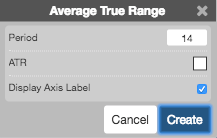Charts

# Average True Range (ATR)

The Average True Range (ATR) study measures the size of the period's range, and takes into account any gap from the close of the previous period.## Configuration Options• Period: Number of bars to use in the calculations.
• Color Selectors: Colors to use for graph elements.
• Display Axis Label: Whether to display the most recent value on the Y axis.

## Formula

$Average\;True\;Range = average(TR, n)$

where:

• $$TR = max(High_{t} - Close_{t-1}\;,\;Close_{t-1} - Low_{t}\;,\;High_{t} - Low_{t})$$
• $$n = period$$